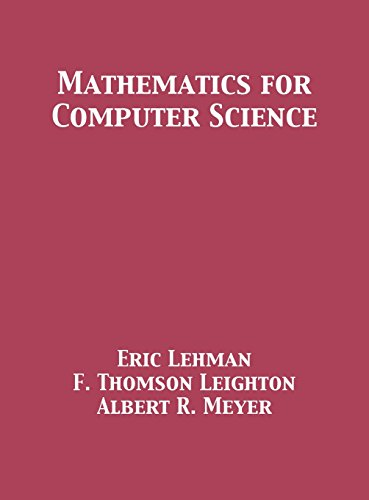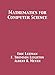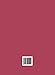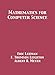Saturday , September 26th 2020

# Mathematics for Computer Science#### Product Features:

This book covers elementary discrete mathematics for computer science and engineering. It emphasizes mathematical definitions and proofs as well as applicable methods. Topics include formal logic notation, proof methods; induction, well-ordering; sets, relations; elementary graph theory; integer congruences; asymptotic notation and growth of functions; permutations and combinations, counting principles;…
Price as on: 2019-11-25 14:44:01

#### Product Description

This book covers elementary discrete mathematics for computer science and engineering. It emphasizes mathematical definitions and proofs as well as applicable methods. Topics include formal logic notation, proof methods; induction, well-ordering; sets, relations; elementary graph theory; integer congruences; asymptotic notation and growth of functions; permutations and combinations, counting principles; discrete probability. Further selected topics may also be covered, such as recursive definition and structural induction; state machines and invariants; recurrences; generating functions.

The color images and text in this book have been converted to grayscale.

## Complexity Theory, Game Theory, and Economics: The

This monograph comprises a series of ten lectures divided into two parts. Part 1, referred to as the Solar…

## Computer Science Education

This book provides an overview of how to approach computer science education research from a pragmatic perspective. It represents…

## Supervised Learning with Quantum Computers (Quantum

Quantum machine learning investigates how quantum computers can be used for data-driven prediction and decision making. The books summarises…